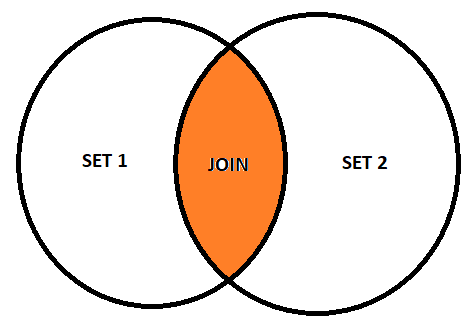# Inner Join SQL

JOIN in SQL are used to combine rows from two or more tables
based on a common field between them, thus returning data from different
tables.

A JOIN occurs when two or more tables are joined in one SQL
statement.

For example, INNER JOIN, Returns all rows when there is at least
one match in both tables.

LEFT JOIN Returns all rows from the left table, and matching rows
from the right table.

RIGHT JOIN Returns all rows from the right table, and matching
rows from the left table.

OUTER JOIN, Returns all the rows of the two tables, the left and
the right. Also called FULL OUTER JOIN.

condition.

## INNER JOIN

The INNER JOIN clause searches for matches between rows in the product
and category tables.

If a row or record in the products table has the same value in the column
as a row in the categories table, the query combines the values ​​of the
columns specified in the SELECT list into a new row and includes
that new row in the result set.

It is the most common type of JOIN.

SELECT columnName(s) FROM table1 INNER JOIN table2 ON
table1.columnName=table2.columnName;

In mathematical terms, it is nothing more than the union of two sets that
have elements in common, so that if we make a diagram, it would look like
this:The example to be used will be in the WideWorldImporters Database,
it will seek to obtain the name of the supplier and the amount of the
transaction, where the IDs in both tables match.

And the following result is obtained:It is also possible to obtain a specific result for each supplier, giving
the identifier number, for example:

Where you can see that you want to obtain the amounts of the transaction
and the corresponding dates, from the supplier identified as 4 (Fabrikam,
Inc.).## INNER JOIN on three Tables

It is also possible to get data from three tables, using the INNER JOIN
clause, twice, as follows:

The following statement uses two INNER JOIN clauses to query data from
all three tables: